# 208114036 l aser guided robo

24 de Jun de 2015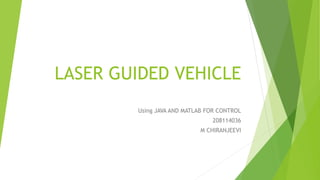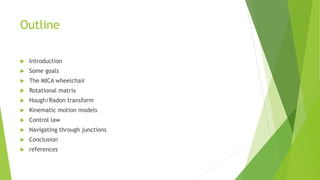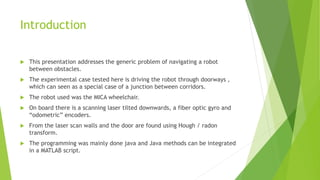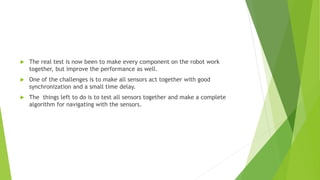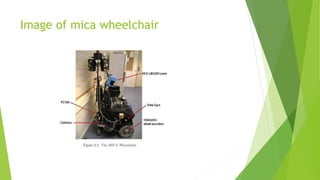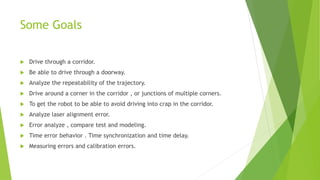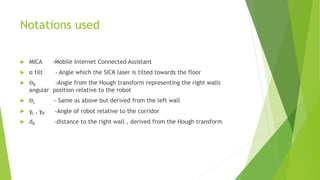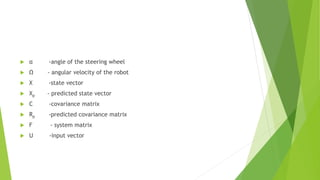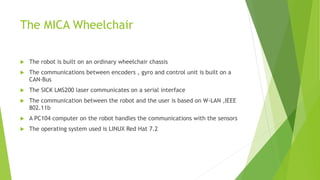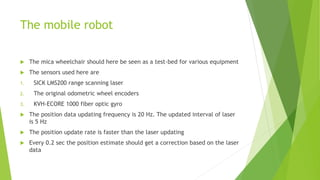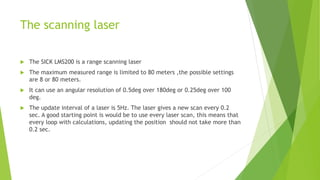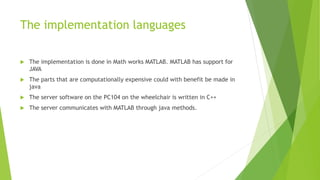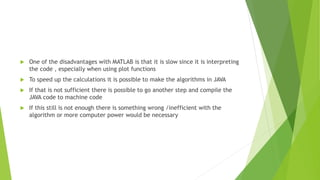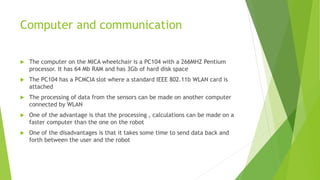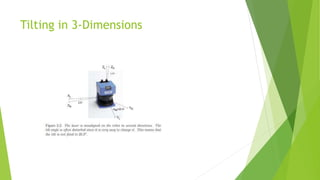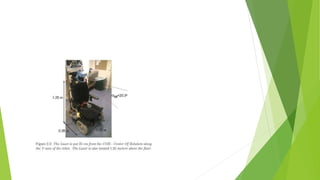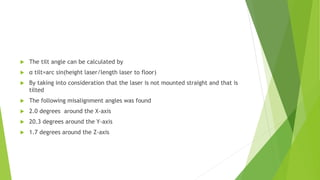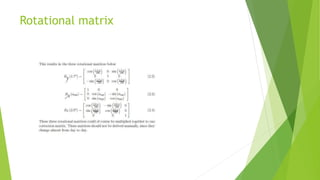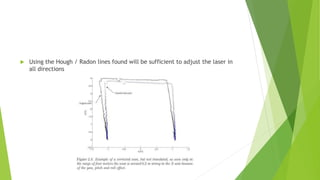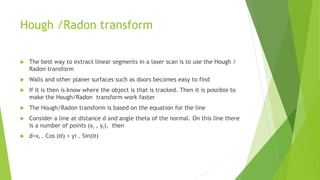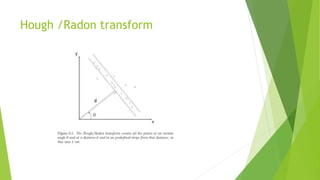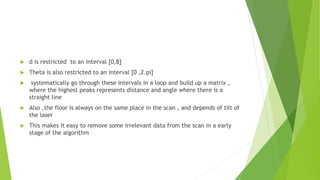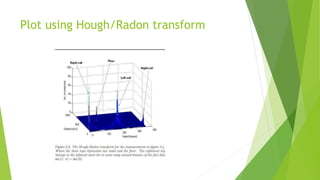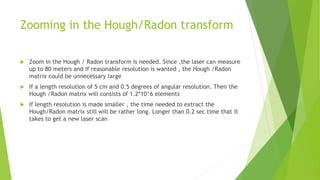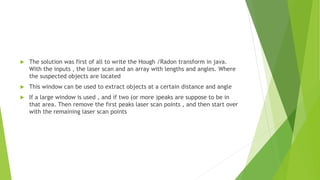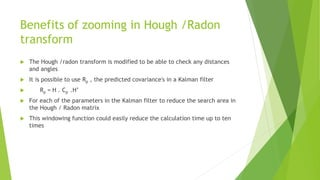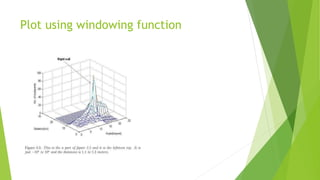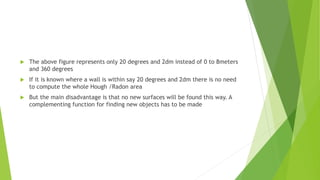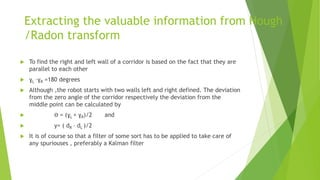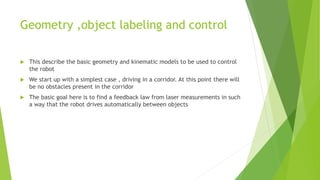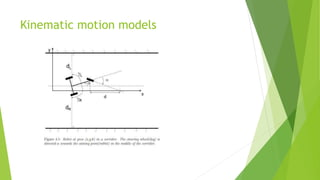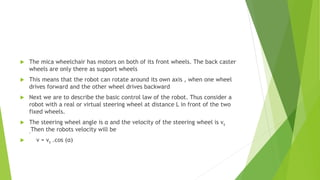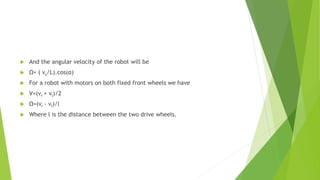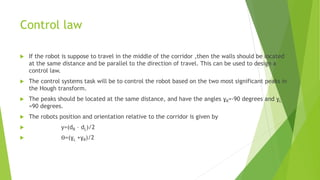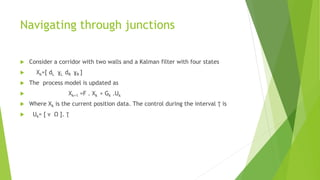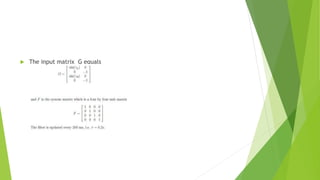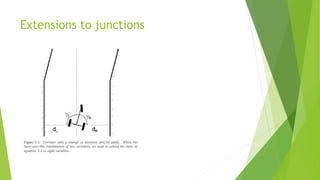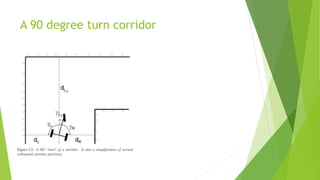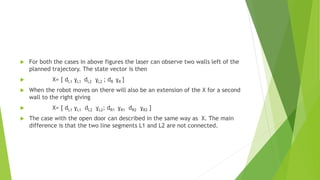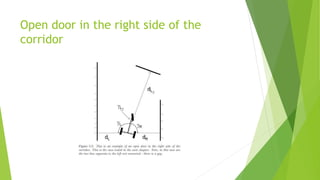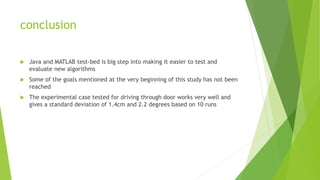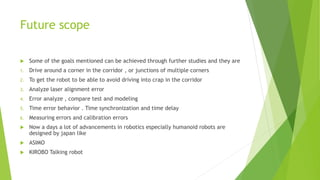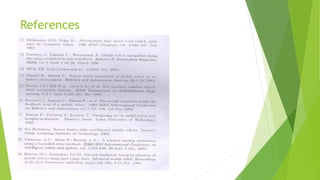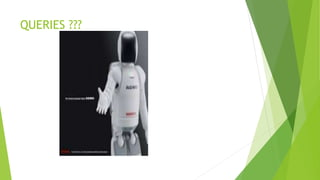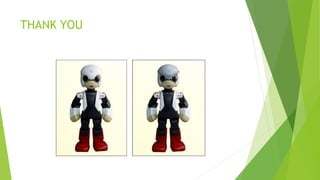1 de 45

### 208114036 l aser guided robo

• 1. LASER GUIDED VEHICLE Using JAVA AND MATLAB FOR CONTROL 208114036 M CHIRANJEEVI
• 2. Outline  Introduction  Some goals  The MICA wheelchair  Rotational matrix  Hough/Radon transform  Kinematic motion models  Control law  Navigating through junctions  Conclusion  references
• 3. Introduction  This presentation addresses the generic problem of navigating a robot between obstacles.  The experimental case tested here is driving the robot through doorways , which can seen as a special case of a junction between corridors.  The robot used was the MICA wheelchair.  On board there is a scanning laser tilted downwards, a fiber optic gyro and “odometric” encoders.  From the laser scan walls and the door are found using Hough / radon transform.  The programming was mainly done java and Java methods can be integrated in a MATLAB script.
• 4.  The real test is now been to make every component on the robot work together, but improve the performance as well.  One of the challenges is to make all sensors act together with good synchronization and a small time delay.  The things left to do is to test all sensors together and make a complete algorithm for navigating with the sensors.
• 5. Image of mica wheelchair
• 6. Some Goals  Drive through a corridor.  Be able to drive through a doorway.  Analyze the repeatability of the trajectory.  Drive around a corner in the corridor , or junctions of multiple corners.  To get the robot to be able to avoid driving into crap in the corridor.  Analyze laser alignment error.  Error analyze , compare test and modeling.  Time error behavior . Time synchronization and time delay.  Measuring errors and calibration errors.
• 7. Notations used  MICA -Mobile Internet Connected Assistant  α tilt - Angle which the SICK laser is tilted towards the floor  ƟR -Angle from the Hough transform representing the right walls angular position relative to the robot  ƟL - Same as above but derived from the left wall  ɣL , ɣR -Angle of robot relative to the corridor  dR -distance to the right wall , derived from the Hough transform
• 8.  α -angle of the steering wheel  Ω - angular velocity of the robot  X -state vector  Xp - predicted state vector  C -covariance matrix  Rp -predicted covariance matrix  F - system matrix  U -input vector
• 9. The MICA Wheelchair  The robot is built on an ordinary wheelchair chassis  The communications between encoders , gyro and control unit is built on a CAN-Bus  The SICK LMS200 laser communicates on a serial interface  The communication between the robot and the user is based on W-LAN ,IEEE 802.11b  A PC104 computer on the robot handles the communications with the sensors  The operating system used is LINUX Red Hat 7.2
• 10. The mobile robot  The mica wheelchair should here be seen as a test-bed for various equipment  The sensors used here are 1. SICK LMS200 range scanning laser 2. The original odometric wheel encoders 3. KVH-ECORE 1000 fiber optic gyro  The position data updating frequency is 20 Hz. The updated interval of laser is 5 Hz  The position update rate is faster than the laser updating  Every 0.2 sec the position estimate should get a correction based on the laser data
• 11. The scanning laser  The SICK LMS200 is a range scanning laser  The maximum measured range is limited to 80 meters ,the possible settings are 8 or 80 meters.  It can use an angular resolution of 0.5deg over 180deg or 0.25deg over 100 deg.  The update interval of a laser is 5Hz. The laser gives a new scan every 0.2 sec. A good starting point is would be to use every laser scan, this means that every loop with calculations, updating the position should not take more than 0.2 sec.
• 12. The implementation languages  The implementation is done in Math works MATLAB. MATLAB has support for JAVA  The parts that are computationally expensive could with benefit be made in java  The server software on the PC104 on the wheelchair is written in C++  The server communicates with MATLAB through java methods.
• 13.  One of the disadvantages with MATLAB is that it is slow since it is interpreting the code , especially when using plot functions  To speed up the calculations it is possible to make the algorithms in JAVA  If that is not sufficient there is possible to go another step and compile the JAVA code to machine code  If this still is not enough there is something wrong /inefficient with the algorithm or more computer power would be necessary
• 14. Computer and communication  The computer on the MICA wheelchair is a PC104 with a 266MHZ Pentium processor. It has 64 Mb RAM and has 3Gb of hard disk space  The PC104 has a PCMCIA slot where a standard IEEE 802.11b WLAN card is attached  The processing of data from the sensors can be made on another computer connected by WLAN  One of the advantage is that the processing , calculations can be made on a faster computer than the one on the robot  One of the disadvantages is that it takes some time to send data back and forth between the user and the robot
• 17.  The tilt angle can be calculated by  α tilt=arc sin(height laser/length laser to floor)  By taking into consideration that the laser is not mounted straight and that is tilted  The following misalignment angles was found  2.0 degrees around the X-axis  20.3 degrees around the Y-axis  1.7 degrees around the Z-axis
• 19.  Using the Hough / Radon lines found will be sufficient to adjust the laser in all directions
• 20. Hough /Radon transform  The best way to extract linear segments in a laser scan is to use the Hough / Radon transform  Walls and other planer surfaces such as doors becomes easy to find  If it is then is know where the object is that is tracked. Then it is possible to make the Hough/Radon transform work faster  The Hough/Radon transform is based on the equation for the line  Consider a line at distance d and angle theta of the normal. On this line there is a number of points (xi , yi), then  d=xi . Cos (Ɵ) + yi . Sin(Ɵ)
• 22.  d is restricted to an interval [0,8]  Theta is also restricted to an interval [0 ,2.pi]  systematically go through these intervals in a loop and build up a matrix , where the highest peaks represents distance and angle where there is a straight line  Also ,the floor is always on the same place in the scan , and depends of tilt of the laser  This makes it easy to remove some irrelevant data from the scan in a early stage of the algorithm
• 24. Zooming in the Hough/Radon transform  Zoom in the Hough / Radon transform is needed. Since ,the laser can measure up to 80 meters and if reasonable resolution is wanted , the Hough /Radon matrix could be unnecessary large  If a length resolution of 5 cm and 0.5 degrees of angular resolution. Then the Hough /Radon matrix will consists of 1.2*10^6 elements  If length resolution is made smaller , the time needed to extract the Hough/Radon matrix still will be rather long. Longer than 0.2 sec time that it takes to get a new laser scan
• 25.  The solution was first of all to write the Hough /Radon transform in java. With the inputs , the laser scan and an array with lengths and angles. Where the suspected objects are located  This window can be used to extract objects at a certain distance and angle  If a large window is used , and if two (or more )peaks are suppose to be in that area. Then remove the first peaks laser scan points , and then start over with the remaining laser scan points
• 26. Benefits of zooming in Hough /Radon transform  The Hough /radon transform is modified to be able to check any distances and angles  It is possible to use Rp , the predicted covariance's in a Kalman filter  Rp = H . Cp .H’  For each of the parameters in the Kalman filter to reduce the search area in the Hough / Radon matrix  This windowing function could easily reduce the calculation time up to ten times
• 28.  The above figure represents only 20 degrees and 2dm instead of 0 to 8meters and 360 degrees  If it is known where a wall is within say 20 degrees and 2dm there is no need to compute the whole Hough /Radon area  But the main disadvantage is that no new surfaces will be found this way. A complementing function for finding new objects has to be made
• 29. Extracting the valuable information from Hough /Radon transform  To find the right and left wall of a corridor is based on the fact that they are parallel to each other  ɣL –ɣR =180 degrees  Although ,the robot starts with two walls left and right defined. The deviation from the zero angle of the corridor respectively the deviation from the middle point can be calculated by  Ɵ = (ɣL + ɣR)/2 and  y= ( dR – dL )/2  It is of course so that a filter of some sort has to be applied to take care of any spuriouses , preferably a Kalman filter
• 30. Geometry ,object labeling and control  This describe the basic geometry and kinematic models to be used to control the robot  We start up with a simplest case , driving in a corridor. At this point there will be no obstacles present in the corridor  The basic goal here is to find a feedback law from laser measurements in such a way that the robot drives automatically between objects
• 32.  The mica wheelchair has motors on both of its front wheels. The back caster wheels are only there as support wheels  This means that the robot can rotate around its own axis , when one wheel drives forward and the other wheel drives backward  Next we are to describe the basic control law of the robot. Thus consider a robot with a real or virtual steering wheel at distance L in front of the two fixed wheels.  The steering wheel angle is α and the velocity of the steering wheel is vs ,Then the robots velocity will be  v = vs .cos (α)
• 33.  And the angular velocity of the robot will be  Ω= ( vs/L).cos(α)  For a robot with motors on both fixed front wheels we have  V=(vr + vl)/2  Ω=(vr - vl)/l  Where l is the distance between the two drive wheels.
• 34. Control law  If the robot is suppose to travel in the middle of the corridor ,then the walls should be located at the same distance and be parallel to the direction of travel. This can be used to design a control law.  The control systems task will be to control the robot based on the two most significant peaks in the Hough transform.  The peaks should be located at the same distance, and have the angles ɣR=-90 degrees and ɣL =90 degrees.  The robots position and orientation relative to the corridor is given by  y=(dR – dL)/2  Ɵ=(ɣL +ɣR)/2
• 35. Navigating through junctions  Consider a corridor with two walls and a Kalman filter with four states  Xk=[ dL ɣL dR ɣR ]  The process model is updated as  Xk+1 =F . Xk + Gk .Uk  Where Xk is the current position data. The control during the interval Ʈ is  Uk= [ v Ω ]. Ʈ
• 36.  The input matrix G equals
• 38. A 90 degree turn corridor
• 39.  For both the cases in above figures the laser can observe two walls left of the planned trajectory. The state vector is then  X= [ dL1 ɣL1 dL2 ɣL2 ; dR ɣR ]  When the robot moves on there will also be an extension of the X for a second wall to the right giving  X= [ dL1 ɣL1 dL2 ɣL2; dR1 ɣR1 dR2 ɣR2 ]  The case with the open door can described in the same way as X. The main difference is that the two line segments L1 and L2 are not connected.
• 40. Open door in the right side of the corridor
• 41. conclusion  Java and MATLAB test-bed is big step into making it easier to test and evaluate new algorithms  Some of the goals mentioned at the very beginning of this study has not been reached  The experimental case tested for driving through door works very well and gives a standard deviation of 1.4cm and 2.2 degrees based on 10 runs
• 42. Future scope  Some of the goals mentioned can be achieved through further studies and they are 1. Drive around a corner in the corridor , or junctions of multiple corners 2. To get the robot to be able to avoid driving into crap in the corridor 3. Analyze laser alignment error 4. Error analyze , compare test and modeling 5. Time error behavior . Time synchronization and time delay 6. Measuring errors and calibration errors  Now a days a lot of advancements in robotics especially humanoid robots are designed by japan like  ASIMO  KIROBO Talking robot